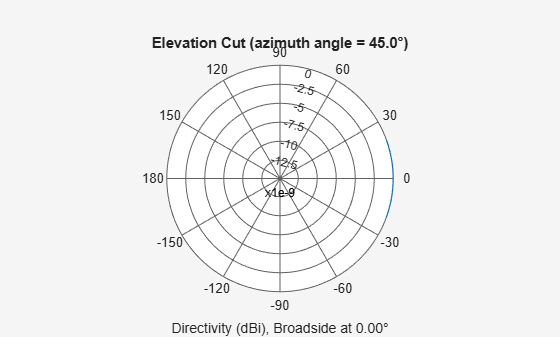# phased.IsotropicProjector

Isotropic projector element

## Description

The `phased.IsotropicProjector` System object™ creates an isotropic sound projector used in sonar applications. An isotropic projector has the same response in all directions. The response is the radiated sound intensity per unit input voltage to the projector. You can change the response using the `VoltageResponse` property.

To compute the response of the isotropic projector for specified directions:

1. Create the `phased.IsotropicProjector` object and set its properties.

2. Call the object with arguments, as if it were a function.

## Creation

### Syntax

``projector = phased.IsotropicProjector``
``projector = phased.IsotropProjector(Name=Value)``

### Description

example

````projector = phased.IsotropicProjector` creates an isotropic projector System object, `projector`.```
````projector = phased.IsotropProjector(Name=Value)` creates an Isotropic projector object, `projector`, with each specified property set to the specified value. You can specify additional name-value pair arguments in any order as (`Name1=Value1`,...,`NameN=ValueN`).Example: ```projector = phased.IsotropicProjector(FrequencyRange=[0 1000],BackBaffled=true)``` creates an isotropic projector element with its frequency range specified between 0 and 1000 Hz.```

## Properties

expand all

Unless otherwise indicated, properties are nontunable, which means you cannot change their values after calling the object. Objects lock when you call them, and the `release` function unlocks them.

If a property is tunable, you can change its value at any time.

Operating frequency range of projector, specified as a 1-by-2 row vector in the form of `[LowerBound HigherBound]`. This property defines the frequency range over which the projector has a response. The projector element has zero response outside this frequency range. Units are in Hz..

Example: `[0 10e3]`

Data Types: `double`

Voltage response of projector, specified as a scalar or real-valued 1-by-K row vector. When you specify voltage response as a scalar, that value applies to the entire frequency range specified by `FrequencyRange`. When you specify the voltage sensitivity as a vector, the frequency range is divided into K-1 equal intervals. The response values are assigned to the interval end points. Then, the `step` method interpolates the voltage response for any frequency inside the frequency range. Units are in dB ref: 1 μPa/V. See Projector Voltage Response for more details.

Example: `10`

Data Types: `double`

Back baffle response of projector, specified as `false` or `true`. Set this property to `true` to back baffle the projector response. When the projector is back baffled, the projector response for all azimuth angles beyond ±90 from broadside are zero. Broadside is defined as 0° azimuth and 0° elevation.

When the value of this property is `false`, the projector is not back baffled.

## Usage

### Syntax

``resp = projector(freq,ang)``

### Description

example

````resp = projector(freq,ang)` returns the voltage response `resp` for the projector at the specified operating frequencies `freq` and in the specified directions `ang` of arriving signals. NoteThe object performs an initialization the first time the object is executed. This initialization locks nontunable properties and input specifications, such as dimensions, complexity, and data type of the input data. If you change a nontunable property or an input specification, the System object issues an error. To change nontunable properties or inputs, you must first call the `release` method to unlock the object. ```

### Input Arguments

expand all

Voltage response frequencies of projector, specified as a positive real scalar or a real-valued 1-by-L vector of positive values. Units are in Hz.

Data Types: `double`

Direction of arriving signals, specified as a real-valued 1-by-M row vector or 2-by-M matrix. When `ang` is a 2-by-M matrix, each column of the matrix specifies the direction in the form `[azimuth;elevation]`. The azimuth angle must lie between –180° and 180°, inclusive. The elevation angle must lie between –90° and 90°, inclusive.

When `ang` is a 1-by-M row vector, each element specifies the azimuth angle of the arriving signal. In this case, the corresponding elevation angle is assumed to be zero.

Data Types: `double`

### Output Arguments

expand all

Voltage response of projector, returned as a real-valued M-by-L matrix. M represents the number of angles specified in `ang`, and L represents the number of frequencies specified in `freq`. Units are in V/Pa.

## Object Functions

To use an object function, specify the System object as the first input argument. For example, to release system resources of a System object named `obj`, use this syntax:

`release(obj)`

expand all

 `beamwidth` Compute and display beamwidth of sensor element pattern `directivity` Directivity of antenna or transducer element `isPolarizationCapable` Antenna element polarization capability `pattern` Plot antenna or transducer element directivity and patterns `patternAzimuth` Plot antenna or transducer element directivity and pattern versus azimuth `patternElevation` Plot antenna or transducer element directivity and pattern versus elevation
 `step` Run System object algorithm `release` Release resources and allow changes to System object property values and input characteristics `reset` Reset internal states of System object

## Examples

collapse all

Find the response of an isotropic projector with default properties. Obtain the voltage response at five different elevation angles: -30°, -15°, 0°, 15° and 30°. All azimuth angles are set to 0°. The voltage response is computed at 2 kHz.

```projector = phased.IsotropicProjector; fc = 2e3; resp = projector(fc,[0,0,0,0,0;-30,-15,0,15,30])```
```resp = 5×1 1 1 1 1 1 ```

Examine the response and patterns of an isotropic projector operating between 1 kHz and 10 kHz.

Set the projector parameters and obtain the voltage response at five different elevation angles: -30°, -15°, 0°, 15° and 30°. All elevation angles at 0° azimuth angle. The voltage response is computed at 2 kHz.

```projector = phased.IsotropicProjector('FrequencyRange',[1,10]*1e3); fc = 2e3; resp = projector(fc,[0,0,0,0,0;-30,-15,0,15,30]);```

Draw a 3-D plot of the voltage response.

```pattern(projector,fc,[-180:180],[-90:90],'CoordinateSystem','polar', ... 'Type','power')```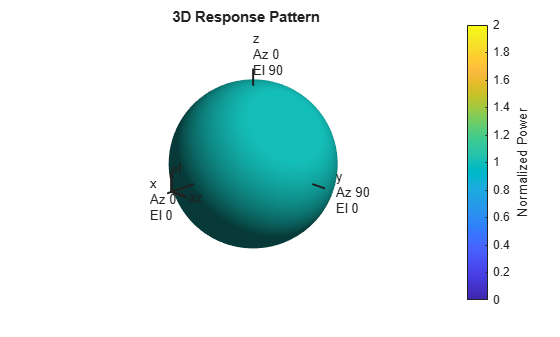Find the beamwidth of an isotropic underwater projector at 10 kHz.

First, create an isotropic projector element.

`projector = phased.IsotropicProjector('FrequencyRange',[10 12000])`
```projector = phased.IsotropicProjector with properties: VoltageResponse: 120 FrequencyRange: [10 12000] BackBaffled: false ```

Then, use the beamwidth object function to plot the 3 dB beamwidth of the projector.

`[bmw,angs] = beamwidth(projector,10000,'dbDown',3)`
```bmw = 360 ```
```angs = 1×2 -180 180 ```

Because the projector is isotropic, there is no 3 dB down point.

Examine the response and patterns of an isotropic projector at three different frequencies. The projector operates between 1 kHz and 10 kHz. Specify the voltage response as a vector.

Set up the projector parameters, and obtain the voltage response at 45° azimuth and 30° elevation. Compute the responses at signal frequencies of 2, 5, and 7 kHz.

```projector = phased.IsotropicProjector('FrequencyRange',[1 10]*1e3, ... 'VoltageResponse',[90 95 100 95 90]); fc = [2e3 5e3 7e3]; resp = projector(fc,[45;30]); resp```
```resp = 1×3 0.0426 0.0903 0.0708 ```

Next, draw a 2-D plot of the voltage response as a function of azimuth.

```pattern(projector,fc,[-180:180],0,'CoordinateSystem','rectangular', ... 'Type','power')```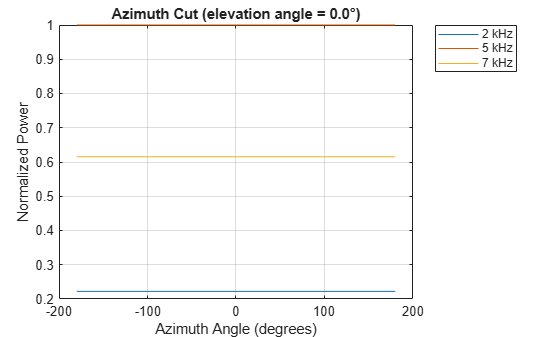Compute the directivity of an isotropic projector in different directions. Assume the signal frequency is 3 kHz. First, set the projector parameters.

```fc = 3e3; projector = phased.IsotropicProjector('FrequencyRange',[1,10]*1e3, ... 'VoltageResponse',[100,110,120,110,100]); patternElevation(projector,fc,45)```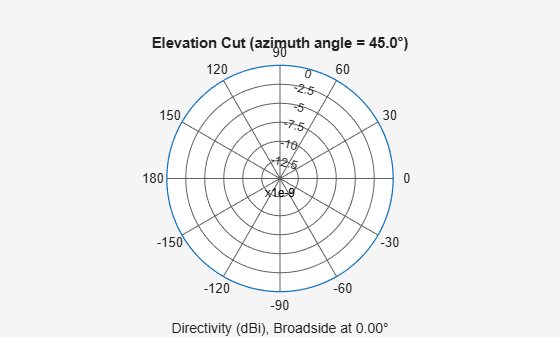Select the angles of interest to be constant elevation angle at zero degrees. The five azimuth angles are centered around boresight (zero degrees azimuth and zero degrees elevation).

`ang = [-20,-10,0,10,20; 0,0,0,0,0];`

Compute the directivity along the constant elevation cut.

`d = directivity(projector,fc,ang)`
```d = 5×1 0 0 0 0 0 ```

The directivity of an isotropic projector is zero in every direction.

Examine the azimuth pattern of an isotropic projector at 30° elevation. The frequency range is between 1 kHz and 10 kHz. Specify the voltage response as a scalar.

Set the projector parameters.

```fc = 3e3; projector = phased.IsotropicProjector('FrequencyRange',[1,10]*1e3, ... 'VoltageResponse',-115); patternAzimuth(projector,fc,30)```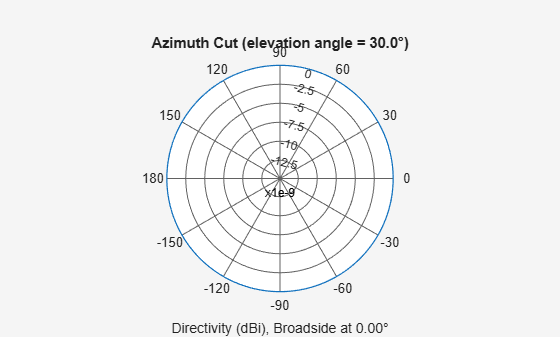Plot a smaller range of azimuth angles using the `Azimuth` parameter.

`patternAzimuth(projector,fc,30,'Azimuth',[-20:20])`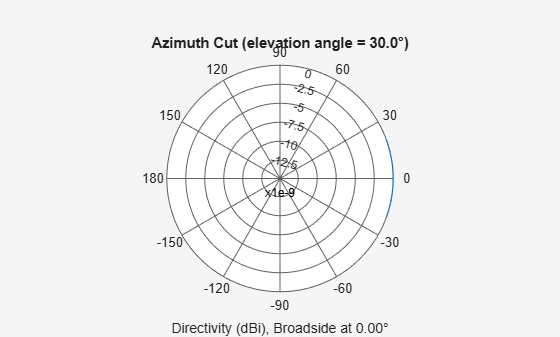Plot an elevation cut of the directivity of an isotropic projector at 45° azimuth. Assume the signal frequency is 3 kHz.

Create the isotropic projector object and call the pattern object function.

```fc = 3e3; projector = phased.IsotropicProjector('FrequencyRange',[1,10]*1e3, ... 'VoltageResponse',70); patternElevation(projector,fc,45)```Plot a smaller range of elevation angles using the `Elevation` parameter.

`patternElevation(projector,fc,45,'Elevation',-20:20)`